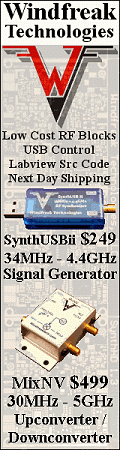Please Support RF Cafe by purchasing my  ridiculously low−priced products, all of which I created.

RF Workbench  (shareware)# Mechanical Units of Measure - Metric & English

Here is a collection of some of the most often used mechanical units. Both SI (metric) and SAE (English) units are given. There are two fundamental types of units: base units and derived units. Base units include meter, foot, kilogram, second, etc. Derived units involve a combination of base units, such as square meter, kilometer/second, etc.

 Name SI Base Unit Symbol English Base Unit Symbol Length meter m foot ft Area square meter m2 square foot ft2 Volume cubic meter m3 cubic foot ft3 Mass kilogram kg pound mass lb Density kilogram/cubic meter kg/m3 pound/cubic foot lb/ft3 Time second s second s Speed kilometer/second km/s foot/second ft/s Force newton N pound-force lbf Pressure pascal P pound/square foot lb/ft2 Plane Angle radian rad degree ° Solid Angle steradian ster square degree deg2 Momentum kilogram*meter/second ρ pound*foot/second ρ Torque newton*meter t pound-force*foot t Young's modulus pascal E pascal E Kinetic energy joule K watt*second K Potential energy joule U watt*second U

Here is the NIST document entitled The International System of Units (SI) –Conversion Factors for General Use